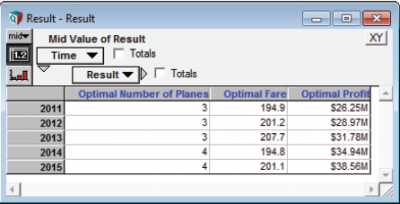# Module 5: Time as an Extrinsic index

Let’s assume that Base Demand increases by 10% per year. How would that affect our decisions over time. In this example, Analytica will abstract over the system index Time instead of Run. The principle is exactly the same as in Module 4.

First, we define the system Time index to represent years from 2011 to 2015. To edit this special index, select Edit Time from the Definition menu and enter this definition:

Index Time := 2011..1015

Starting with the Base Case setup, create a new variableGrowth_Rate. Re-define Base_Demand so that it grows exponentially over time using this annual growth rate.

Variable Growth_Rate := 10%
Variable Base_Demand := 400k*(1 + Growth_Rate)^(@Time - 1)

This definition uses a positional reference for the Time index. The first year corresponds to position 1 (making the exponent zero). Thus, Base Demand for the first year will be 400k, and this value increases 10% every year.

Time is now an index of Base Demand. The extrinsic index is propagated to the Objective. Array abstraction results in a separate optimization for each year.Profit rises in each year as demand increases. A new plane is added in 2014. Wait! Did you forget something? This in an NLP with an extrinsic index. If you remembered to set a context variable you win a free Grande Analyti-latte! Base_Demand is the sensible choice.

Variable Opt := DefineOptimization(
Decisions: Number_of_Planes, Fare,
Maximize: Profit,
SetContext: Base_Demand)